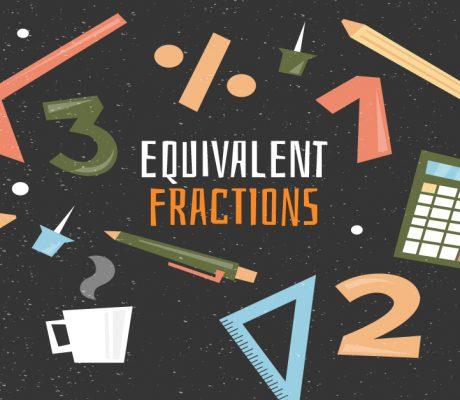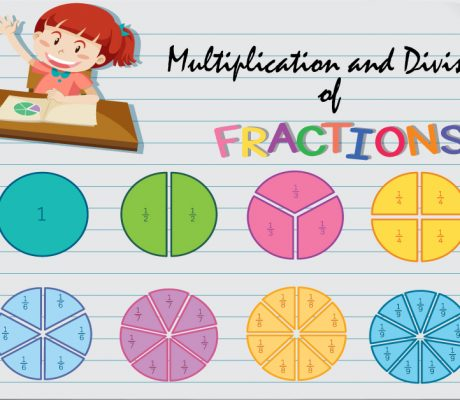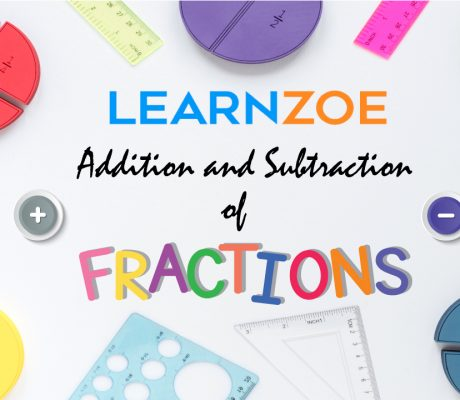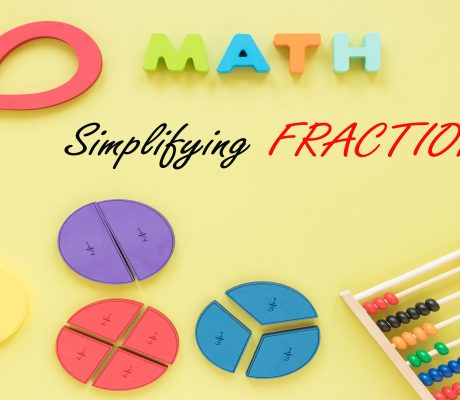# Category: Grade 4 Lessons### Equivalent Fractions

Equivalent fractions are fractions that represent the same size even if the numbers look different. Let’s take a look at the pizzas below. The two fractions have different numerators and denominators, but they represent the same size of pizza slices. when simplified, is actually . It is important to remember that these two fractions still […]### Multiplying and Dividing Fractions

The truth is multiplying and dividing fractions are easier than adding and subtracting fractions. It does not require a lot of steps because it only involves multiplying and simplifying.  When multiplying fractions, you take the numerators and multiply them. You also do the same with the denominators. If needed, the final product is then simplified […]### Learn ZOE Math Practice Questions with Answers

In this blog, there are 21 math practice questions that Learn ZOE teachers prepared for you. The questions are based on different grade levels and once the answer is revealed, there will be a detailed explanation of how we got the correct answer. Feel free to answer the math practice questions first before revealing the […]### Adding and Subtracting Fractions

Adding and Subtracting fractions may seem a terrifying Math lesson for some, but the truth is it is not as complicated as some people might think. The trick is to be able to recognize and translate fractions into familiar objects; which is why pizza slices and chocolate bars are commonly used to represent fractions. This […]